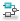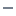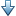Main Content

## Weighted Gain Goal

### Purpose

Frequency-weighted gain limit for tuning with Control System Tuner.

### Description

Weighted Gain Goal limits the gain of the frequency-weighted transfer function WL(s)H(s)WR(s), where H(s) is the transfer function between inputs and outputs you specify. WL(s) and WR(s) are weighting functions that you can use to emphasize particular frequency bands. Weighted Gain Goal constrains the peak gain of WL(s)H(s)WR(s) to values less than 1. If H(s) is a MIMO transfer function, Weighted Gain Goal constrains the largest singular value of H(s).

By default, Weighted Gain Goal constrains a closed-loop gain. To constrain a gain computed with one or more loops open, specify loop-opening locations in the I/O Transfer Selection section of the dialog box.

#### Creation

In the Tuning tab of Control System Tuner, select New Goal > Frequency-weighted gain limit to create a Weighted Gain Goal.

#### Command-Line Equivalent

When tuning control systems at the command line, use `TuningGoal.WeightedGain` to specify a weighted gain goal.

### I/O Transfer Selection

Use this section of the dialog box to specify the inputs and outputs of the transfer function that the tuning goal constrains. Also specify any locations at which to open loops for evaluating the tuning goal.

• Specify input signals

Select one or more signal locations in your model as inputs to the transfer function that the tuning goal constrains. To constrain a SISO response, select a single-valued input signal. For example, to constrain the gain from a location named `'u'` to a location named `'y'`, clickAdd signal to list and select `'u'`. To constrain the largest singular value of a MIMO response, select multiple signals or a vector-valued signal.

• Specify output signals

Select one or more signal locations in your model as outputs of the transfer function that the tuning goal constrains. To constrain a SISO response, select a single-valued output signal. For example, to constrain the gain from a location named `'u'` to a location named `'y'`, clickAdd signal to list and select `'y'`. To constrain the largest singular value of a MIMO response, select multiple signals or a vector-valued signal.

• Compute input/output gain with the following loops open

Select one or more signal locations in your model at which to open a feedback loop for the purpose of evaluating this tuning goal. The tuning goal is evaluated against the open-loop configuration created by opening feedback loops at the locations you identify. For example, to evaluate the tuning goal with an opening at a location named `'x'`, clickAdd signal to list and select `'x'`.

Tip

To highlight any selected signal in the Simulink® model, click. To remove a signal from the input or output list, click. When you have selected multiple signals, you can reorder them usingand. For more information on how to specify signal locations for a tuning goal, see Specify Goals for Interactive Tuning.

### Weights

Use the Left weight WL and Right weight WR text boxes to specify the frequency-weighting functions for the tuning goal. The tuning goal ensures that the gain H(s) from the specified input to output satisfies the inequality:

||WL(s)H(s)WR(s)|| < 1.

WL provides the weighting for the output channels of H(s), and WR provides the weighting for the input channels. You can specify scalar weights or frequency-dependent weighting. To specify a frequency-dependent weighting, use a numeric LTI model whose magnitude represents the desired weighting function. For example, enter `tf(1,[1 0.01])` to specify a high weight at low frequencies that rolls off above 0.01 rad/s.

If the tuning goal constrains a MIMO transfer function, scalar or SISO weighting functions automatically expand to any input or output dimension. You can specify different weights for each channel by specifying matrices or MIMO weighting functions. The dimensions H(s) must be commensurate with the dimensions of WL and WR. For example, if the constrained transfer function has two inputs, you can specify `diag([1 10])` as WR.

If you are tuning in discrete time, you can specify the weighting functions as discrete-time models with the same sampling time as you use for tuning. If you specify the weighting functions in continuous time, the tuning software discretizes them. Specifying the weighting functions in discrete time gives you more control over the weighting functions near the Nyquist frequency.

### Options

Use this section of the dialog box to specify additional characteristics of the weighted gain goal.

• Stabilize I/O transfer

By default, the tuning goal imposes a stability requirement on the closed-loop transfer function from the specified inputs to outputs, in addition to the gain constraint. If stability is not required or cannot be achieved, select `No` to remove the stability requirement. For example, if the gain constraint applies to an unstable open-loop transfer function, select `No`.

• Enforce goal in frequency range

Limit the enforcement of the tuning goal to a particular frequency band. Specify the frequency band as a row vector of the form `[min,max]`, expressed in frequency units of your model. For example, to create a tuning goal that applies only between 1 and 100 rad/s, enter `[1,100]`. By default, the tuning goal applies at all frequencies for continuous time, and up to the Nyquist frequency for discrete time.

• Apply goal to

Use this option when tuning multiple models at once, such as an array of models obtained by linearizing a Simulink model at different operating points or block-parameter values. By default, active tuning goals are enforced for all models. To enforce a tuning requirement for a subset of models in an array, select Only Models. Then, enter the array indices of the models for which the goal is enforced. For example, suppose you want to apply the tuning goal to the second, third, and fourth models in a model array. To restrict enforcement of the requirement, enter `2:4` in the Only Models text box.

For more information about tuning for multiple models, see Robust Tuning Approaches (Robust Control Toolbox).

### Algorithms

When you tune a control system, the software converts each tuning goal into a normalized scalar value f(x). Here, x is the vector of free (tunable) parameters in the control system. The software then adjusts the parameter values to minimize f(x) or to drive f(x) below 1 if the tuning goal is a hard constraint.

For Weighted Gain Goal, f(x) is given by:

`$f\left(x\right)={‖WL\text{\hspace{0.17em}}H\left(s,x\right)\text{\hspace{0.17em}}\text{\hspace{0.17em}}WR‖}_{\infty }.$`

H(s,x) is the closed-loop transfer function between the specified inputs and outputs, evaluated with parameter values x. Here, ${‖\text{\hspace{0.17em}}\cdot \text{\hspace{0.17em}}‖}_{\infty }$ denotes the H norm (see `getPeakGain`).

This tuning goal also imposes an implicit stability constraint on the weighted closed-loop transfer function between the specified inputs to outputs, evaluated with loops opened at the specified loop-opening locations. The dynamics affected by this implicit constraint are the stabilized dynamics for this tuning goal. The Minimum decay rate and Maximum natural frequency tuning options control the lower and upper bounds on these implicitly constrained dynamics. If the optimization fails to meet the default bounds, or if the default bounds conflict with other requirements, on the Tuning tab, use Tuning Options to change the defaults.

## Support

#### Learn how to automatically tune PID controller gains

Download code examples